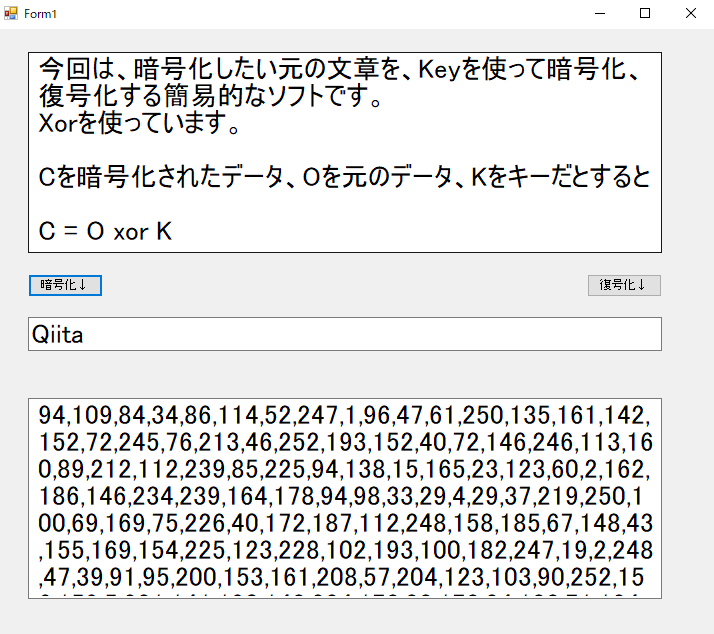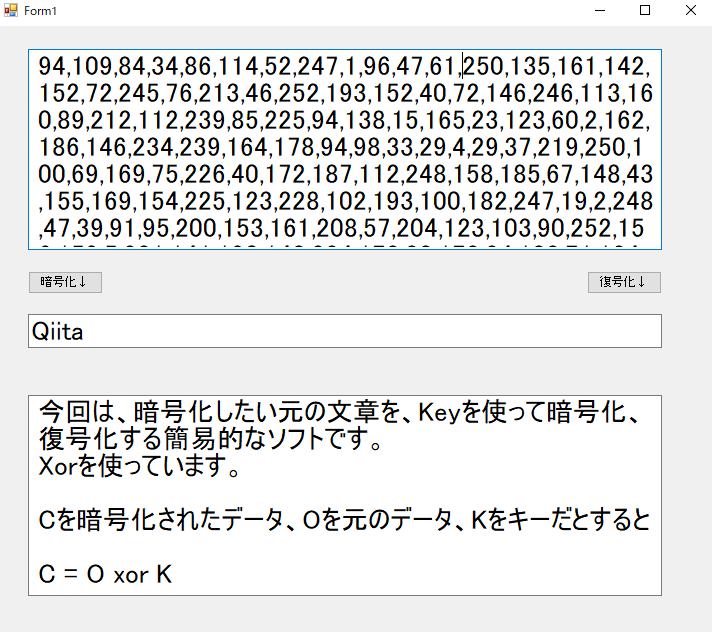# I made a simple encryption software in C #.

This time, it is a simple software that encrypts and decrypts the original text you want to encrypt using Key.
I’m using Xor.

If C is the encrypted data, O is the original data, and K is the key

C = O xor K

And encrypt

O = C xor K

You can decrypt it with.
It is an encryption that uses the nature of this Xor.

## program

It’s organized into classes, so you can make it into a library or embed it.

#### `code.cs`

``````
using System;
using System.Collections.Generic;
using System.Linq;
using System.Text;

namespace Ango
{
class code
{
public string encrypt(string original,string key)
{
string str = original;
byte[] arr = Encoding.GetEncoding("Shift_JIS").GetBytes(str);
string str2 = key;
byte[] arr2 = Encoding.GetEncoding("Shift_JIS").GetBytes(str2);

string out_string = "";
int str2_index = 0;
int str2_next_index;

for (int i = 0; i < arr.Length; i++)
{
str2_next_index = str2_index + 1;
if (str2_next_index >= arr2.Length) str2_next_index = 0;

arr2[str2_index] = (byte)((arr2[str2_index] + arr2[str2_next_index]) ^ arr2[str2_next_index]);
arr2[str2_next_index] = (byte)(arr2[str2_index] + arr2[str2_next_index]);

out_string = out_string + (arr[i] ^ arr2[str2_index]).ToString() + ",";

str2_index++;
if (str2_index >= arr2.Length) str2_index = 0;
}

return out_string;
}

public string decrypt(string cryptogram,string key)
{
string[] arr = cryptogram.Split(',');
byte[] byte_arr = new byte[arr.Length];

string str2 = key;
byte[] arr2 = Encoding.GetEncoding("Shift_JIS").GetBytes(str2);

int str2_index = 0;
int str2_next_index;

for (int i = 0; i < arr.Length - 1; i++)
{
str2_next_index = str2_index + 1;
if (str2_next_index >= arr2.Length) str2_next_index = 0;

arr2[str2_index] = (byte)((arr2[str2_index] + arr2[str2_next_index]) ^ arr2[str2_next_index]);
arr2[str2_next_index] = (byte)(arr2[str2_index] + arr2[str2_next_index]);

byte_arr[i] = Convert.ToByte(arr[i]);
byte_arr[i] = (byte)(byte_arr[i] ^ arr2[str2_index]);

str2_index++;
if (str2_index >= arr2.Length) str2_index = 0;
}

string out_str = Encoding.GetEncoding("Shift_JIS").GetString(byte_arr);

return out_str;
}
}
}
``````

## Commentary

The function ʻencrypt` encrypts.
Pass the original text and key as arguments.
The encrypted version is returned in text format.

First, change the original text and key from `String` to` Byte [] `.
Xor while shifting the two `Byte []` by 1 byte.

With this alone, it seems that the key is easy to guess, so I am constantly changing the key.

``````                arr2[str2_index] = (byte)((arr2[str2_index] + arr2[str2_next_index]) ^ arr2[str2_next_index]);
arr2[str2_next_index] = (byte)(arr2[str2_index] + arr2[str2_next_index]);
``````

The function `decrypt` is a function to decrypt.
Pass the encrypted text and key as arguments.
The decrypted text is returned.

The rest is almost the same as when encrypting.

## Example of use``````135,168,181,167,173,90,27,45,183,0,220,38,143,140,145,252,201,93,82,34,227,101,95,234,150,153,76,118,94,33,1,93,20,74,41,192,184,27,143,147,163,76,95,170,199,9,222,177,50,45,233,232,244,49,173,221,229,158,220,65,132,217,75,38,221,150,20,152,149,2,64,172,236,151,155,98,78,22,237,202,107,47,40,205,107,160,67,237,166,173,235,46,249,188,154,9,241,140,222,211,209,101,136,149,175,224,113,128,100,233,145,140,153,104,201,230,10,165,140,115,161,124,85,76,206,185,67,158,7,201,153,124,44,29,200,170,132,29,145,4,228,15,230,139,19,78,71,91,193,93,225,154,